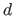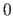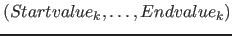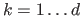Next: Project structur of the Up: Fib elements Previous: Set-element   Contents   Index

### Matrix element

For the description of the matrix element see section 9.13 on page.

The name of the XML element is `matrix`. It has the attribute `dimensions`, which indicates how many dimensionsthe matrix element has. Furthermore it has an optional attribute named `domainNr`, which specifies the number of the domain for the matrix element. If the attribute `domainNr` is missing, the domain number is.

The element contains first a XML element named `defined_variables`, wich contains for each variable, which the matrix element defines, in their order a `variable` element. These have the optional attribute `number`, which is the number (counting begins at) of the element respectively variable defined in the matrix element. Furthermore the `variable` element contains a natural number / identifier for the variable, that is not used above the matrix element for another variable.

After the XML element for variable definitions `defined_variables` follows the XML element named `areas`, wich contains the vectors with the areas for the dimension (with), to which the dimension variables () should be set to. They are listed in their order in the matrix element. The vectors all have the type `area` (`type=ärea"`).

After the XML element for the areas `areas` follows the XML element named `values`, wich contains the vectors with the values (), to which the variables should be set. They are listed in their order in the matrix element. The vectors all have the type `matrix` (`type="matrix"`).

At the end, after the XML element for the values vectors `values`, the matrix element contains the XML element for the contained subobject.

An example for a matrix element:

```<matrix dimensions="2">
<defined_variables>
<variable>7</variable>
<variable>8</variable>
<variable>9</variable>
<variable>10</variable>
<variable>11</variable>
</defined_variables>
<areas>
<vector type=ärea">
<value>3</value>
<value>4</value>
</vector>
<vector type=ärea">
<variable>2</variable>
<value>7</value>
</vector>
</areas>
<values>
<vector type="matrix">
<variable>1</variable>
<value>3</value>
<value>26.14</value>
</vector>
<vector type="matrix">
<value>33.4</value>
<value>-47</value>
<variable>4</variable>
</vector>
</values>
...
...<!-- Fib subobject -->
</matrix>
```

An example for a matrix element with the optional attribute `domainNr` and `number`:

```<matrix dimensions="2" domainNr="7">
<defined_variables>
<variable number="1">8</variable>
<variable number="2">9</variable>
<variable number="3">10</variable>
<variable number="4">11</variable>
</defined_variables>
<areas>
<vector type=ärea">
<variable>3</variable>
<variable>2</variable>
</vector>
<vector type=ärea">
<value>-2</value>
<value>0</value>
</vector>
</areas>
<values>
<vector type="matrix">
<value>3</value>
<variable>1</variable>
</vector>
<vector type="matrix">
<value>0.44</value>
<value>-7</value>
</vector>
<vector type="matrix">
<value>6</value>
<value>5</value>
</vector>
<vector type="matrix">
<value>-1</value>
<value>-1</value>
</vector>
</values>
...
...<!-- Fib subobject -->
</matrix>
```Next: Project structur of the Up: Fib elements Previous: Set-element   Contents   Index
Betti Österholz 2013-02-13